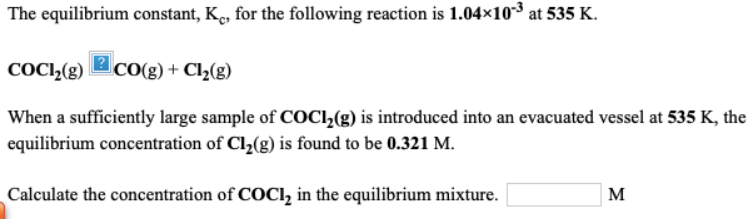# The equilibrium constant, Kc, for the following reaction is 1.04 x 10^-3 at 535 K. COCl2(g) -> CO(g) + Cl2(g) When a sufficiently large sample of COCl2(g) is introduced into an evacuated vessel at 535 K, the equilibrium concentration of Cl2(g) is found to be 0.321 M. Calculate the concentration of COCL2 in the equilibrium mixture. ___ M